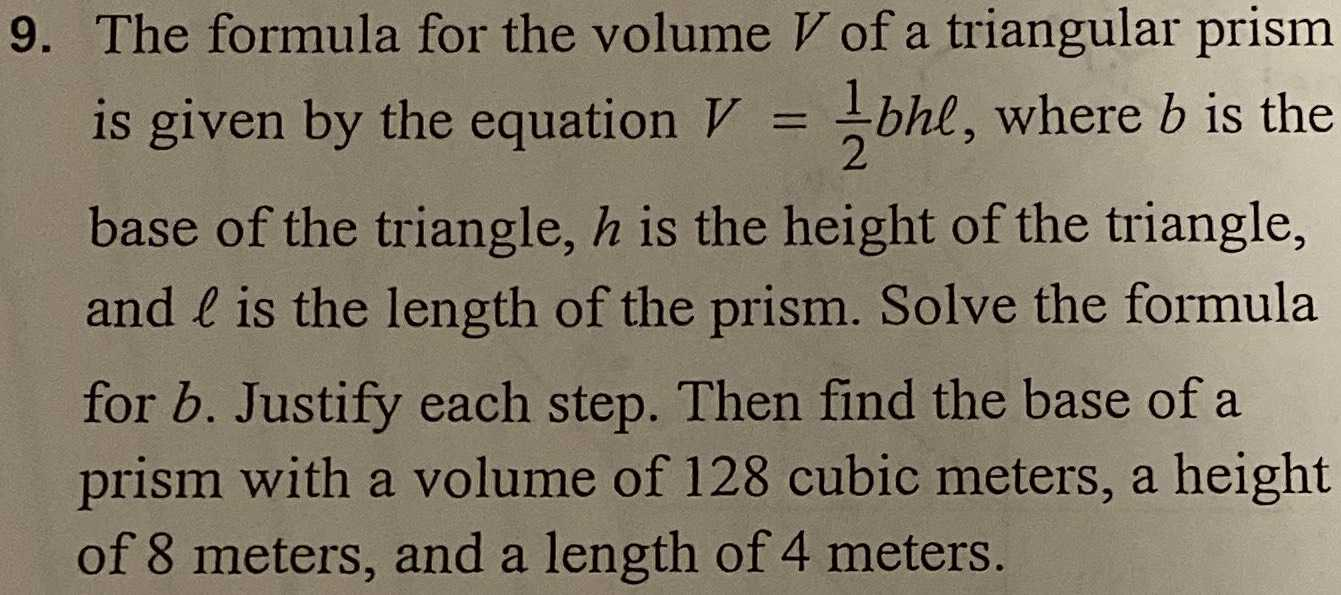### ¿Todavía tienes preguntas de matemáticas?

Pregunte a nuestros tutores expertos
Algebra
Pregunta9. The formula for the volume $$V$$ of a triangular prism is given by the equation $$V = \frac { 1 } { 2 } b h \ell$$ , where $$b$$ is the base of the triangle, $$h$$ is the height of the triangle, and $$\ell$$ is the length of the prism. Solve the formula for $$b$$ . Justify each step. Then find the base of a prism with a volume of $$128$$ cubic meters, a height of $$8$$ meters, and a length of $$4$$ meters.